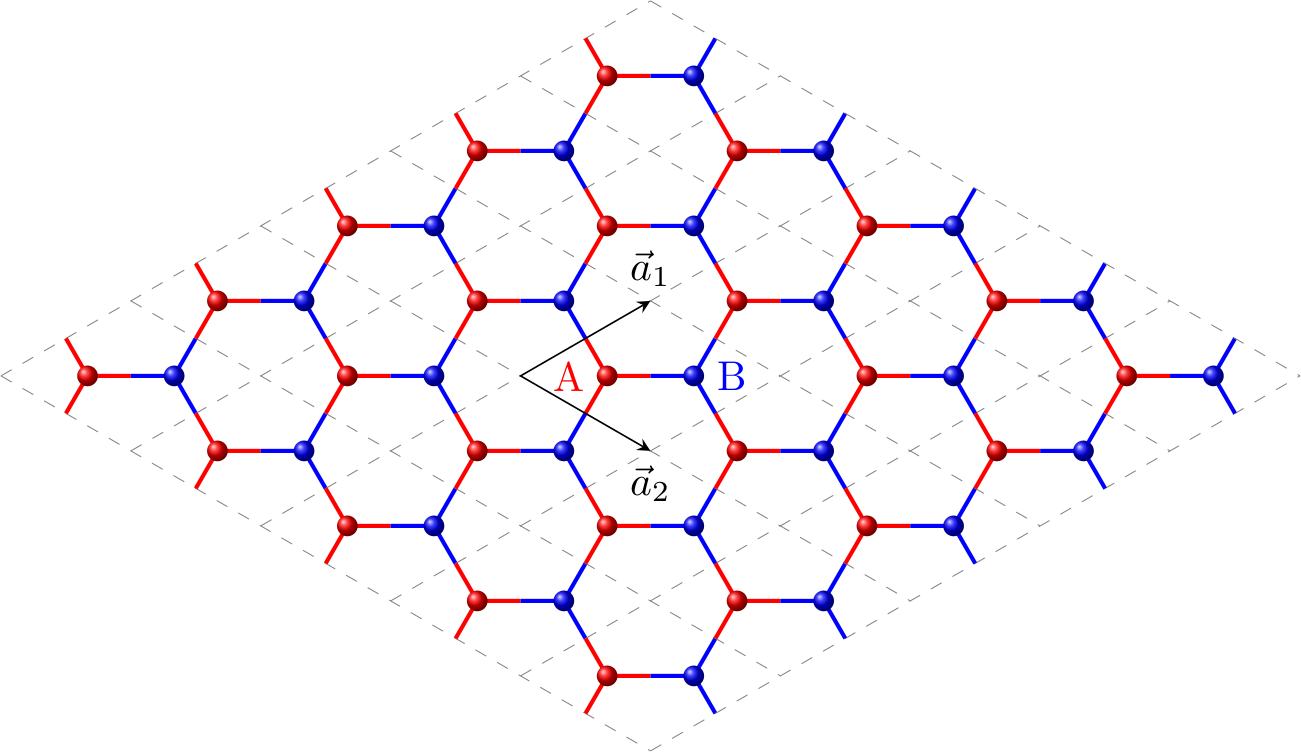# 紧束缚模型与Wannier函数介绍

$\psi_{𝐤n}(𝐫)=𝚎^{𝚒𝐤\cdot 𝐫}u_{𝐤n}(𝐫)$

$u_{𝐤n}(𝐫+\text{任意晶格矢量}𝐑)=u_{𝐤n}(𝐫).$

(1)

$\left|\psi_{𝐑n}\right>\equiv\frac{1}{\sqrt N}\sum_𝐤^{\text{B.Z.}}𝚎^{-𝚒𝐤\cdot 𝐑}\left|\psi_{𝐤n}\right>$

(2)

$\left|\psi_{𝐤n}\right>\equiv\frac{1}{\sqrt N}\sum_𝐑𝚎^{𝚒𝐤\cdot 𝐑}\left|\psi_{𝐑n}\right>$

$\hat{H}_0=\frac{\hat{𝐩}^2}{2m}+V(𝐫),$

(3)

$\hat{H}_0=\int\mathrm{d}\,𝐫\,a^{\dagger}_\sigma(𝐫)\left[\frac{\hat{𝐩}^2}{2m}+V(𝐫)\right]a_\sigma(𝐫),$

$\left|\psi_{𝐤n}\right> =a^\dagger_{𝐤\sigma}\left|0\right>,$

(4)

$\hat{H}_0=\sum_{𝐤}\epsilon_ka^\dagger_{𝐤\sigma}a_{𝐤\sigma}.$

(5)

$a^\dagger_{𝐤\sigma}=\frac{1}{\sqrt N}\sum_𝐑𝚎^{𝚒𝐤\cdot 𝐑_i}a^\dagger_{i\sigma}, \quad a^\dagger_{i\sigma}=\frac{1}{\sqrt N}\sum_𝐤^{\text{B.Z.}}𝚎^{-𝚒𝐤\cdot 𝐑_i}a^\dagger_{𝐤\sigma}$

(6)

$\hat{H}_0=\frac{1}{N}\sum_{ii'}\sum_{𝐤}𝚎^{𝚒𝐤\cdot (𝐑_i-𝐑_{i'})}\epsilon_ka^\dagger_{i\sigma}a_{i'\sigma}\equiv\sum_{ii'}a^\dagger_{i\sigma}t_{ii'}a_{i'\sigma},$

1. 对于$i=i'$也就对同一位置的电子，哈密顿量的对角位，$t_{ii}=\frac{\sum_𝐤\epsilon_k}{N}\equiv \epsilon$，理解上大致对应一个局域电子本身的能量；

2. $i\neq i'$最近邻电子之间，哈密顿量的非对角位，计算得到的$t_{ii'}=N^{-1}\sum_{ii'}\sum_{𝐤}𝚎^{𝚒𝐤\cdot (𝐑_i-𝐑_{i'})}\epsilon_k$一般为一个负值（在紧束缚近似下，$t_{ii'}=-t,\,t>0$），理解为局域的电子向其最近邻位置有一定跳跃的可能，这种跳跃增加了局域电子的活动空间，从而降低了系统的能量。
这里大家可能有点迷糊：$t_{ii'}=N^{-1}\sum_{ii'}\sum_{𝐤}𝚎^{𝚒𝐤\cdot (𝐑_i-𝐑_{i'})}\epsilon_k$这个公式看起来非常的不直观，不知道它代表了什么样的物理意义，怎么就莫名奇妙约等于一个负的常数$-t$了呢？如果我们换一种写法，不是用(4)的从布洛赫态展开哈密顿量，而是用(3)的更为一般的实坐标态下的哈密顿量写，就会更清楚。我们使用$a^{\dagger}_\sigma(𝐫)\rightarrow a^\dagger_{i\sigma}$的表象变换（推导过程与(5)原理一致）：

$a^{\dagger}_\sigma(𝐫)=\sum_i \psi_{𝐑_i}^*(𝐫)a^\dagger_{i\sigma}$

将(3)变为：

$\hat{H}_0=\sum_{ii'} \int \mathrm{d}\,𝐫\,\psi_{𝐑_i}^*(𝐫)a^\dagger_{i\sigma}\left[\frac{\hat{𝐩}^2}{2m}+V(𝐫)\right]\psi_{𝐑_{i'}}(𝐫)a_{i'\sigma},$

也就是：$t_{ii'}=\left<\psi_{𝐑_i}\right|\hat{H}_0\left|\psi_{𝐑_{i'}}\right>=t_{i'i}^*$。这种量子力学中的矩阵元大家应该熟悉，体现的是最近邻两个Wannier函数的重合部分对能量的贡献，也应该理解为什么我们自然而然将它近似为常数。

(7)

$t_{ii'}=\left\{\begin{array}{ll}\epsilon & i=i'\\-t & i\,i' \text{ nearest neighbours}\\0 & \text{otherwise}\end{array}\right.$

# 石墨烯结构This figure is generated by TikZ/LaTeX（From郑奇靖博文

1. 向右连接本晶胞内$𝐑(0,0)$的B，
2. 向左上连接晶胞$𝐑(0,-1)$中的B，
3. 向左下连接晶胞$𝐑(-1,0)$中的B。

1. 向左连接本晶胞内$𝐑(0,0)$的A，
2. 向右下连接晶胞$𝐑(0,1)$中的A，
3. 向右上连接晶胞$𝐑(1,0)$中的A。

# 紧束缚近似应用于石墨烯𝜋键

(8)

\begin{aligned} \hat{H}_\text{diag}&=\sum_i\epsilon(a^\dagger_{i1\sigma}a_{i1\sigma}+a^\dagger_{i2\sigma}a_{i2\sigma})\\ &=\epsilon\sum_i\sum_{𝐤𝐤'}\frac{1}{N}𝚎^{-𝚒𝐤\cdot 𝐑_i}a^\dagger_{𝐤1\sigma}𝚎^{𝚒𝐤'\cdot 𝐑_i}a_{𝐤'1\sigma} + (1\rightarrow 2)\\ &=\epsilon\sum_{𝐤𝐤'}\sum_i\frac{1}{N}𝚎^{𝚒(𝐤'-𝐤)\cdot 𝐑_i}a^\dagger_{𝐤1\sigma}a_{𝐤'1\sigma} + (1\rightarrow 2)\\ &=\epsilon\sum_{𝐤𝐤'}\delta(𝐤'-𝐤)a^\dagger_{𝐤1\sigma}a_{𝐤'1\sigma} + (1\rightarrow 2)\\ &=\epsilon\sum_{𝐤}a^\dagger_{𝐤1\sigma}a_{𝐤1\sigma} + (1\rightarrow 2) \end{aligned}

(9)

\begin{aligned} \hat{H}_\text{offdiag}=& \sum_i -t(a^\dagger_{i1\sigma}a_{i2\sigma} +a^\dagger_{i1\sigma}a_{(𝐑_i+𝐑(0,-1))2\sigma} +a^\dagger_{i1\sigma}a_{(𝐑_i+𝐑(-1,0))2\sigma} ) \\ &+\sum_i -t(a^\dagger_{i2\sigma}a_{i1\sigma} +a^\dagger_{i2\sigma}a_{(𝐑_i+𝐑(0,1))1\sigma} +a^\dagger_{i2\sigma}a_{(𝐑_i+𝐑(1,0))1\sigma} ) \\ =& -t\sum_i\sum_{𝐤𝐤'}\frac{1}{N}\left[𝚎^{𝚒(𝐤'-𝐤)\cdot 𝐑_i}+𝚎^{𝚒[𝐤'\cdot(𝐑_i-𝐚_2)-𝐤\cdot 𝐑_i]}+𝚎^{𝚒[𝐤'\cdot(𝐑_i-𝐚_1)-𝐤\cdot 𝐑_i]}\right]a^\dagger_{𝐤1\sigma}a_{𝐤'2\sigma} +\text{h.c.}\\ =& -t\sum_{𝐤𝐤'}\sum_i\frac{1}{N}𝚎^{𝚒(𝐤'-𝐤)\cdot 𝐑_i}\left(1+𝚎^{-𝚒𝐤'\cdot𝐚_2}+𝚎^{-𝚒𝐤'\cdot𝐚_1}\right)a^\dagger_{𝐤1\sigma}a_{𝐤'2\sigma} +\text{h.c.}\\ =& -t\sum_{𝐤𝐤'}\delta(𝐤'-𝐤)\left(1+𝚎^{-𝚒𝐤'\cdot𝐚_2}+𝚎^{-𝚒𝐤'\cdot𝐚_1}\right)a^\dagger_{𝐤1\sigma}a_{𝐤'2\sigma} +\text{h.c.}\\ =& -t\sum_{𝐤}\left(1+𝚎^{-𝚒𝐤\cdot𝐚_2}+𝚎^{-𝚒𝐤\cdot𝐚_1}\right)a^\dagger_{𝐤1\sigma}a_{𝐤2\sigma} +\text{h.c.} \end{aligned}

(10)

$\hat{H}(𝐤)=\begin{bmatrix}a^\dagger_{𝐤1\sigma} & a^\dagger_{𝐤2\sigma}\end{bmatrix} \begin{bmatrix}\epsilon & -t\left(1+𝚎^{-𝚒𝐤\cdot𝐚_2}+𝚎^{-𝚒𝐤\cdot𝐚_1}\right)\\ -t\left(1+𝚎^{𝚒𝐤\cdot𝐚_2}+𝚎^{𝚒𝐤\cdot𝐚_1}\right) & \epsilon \end{bmatrix} \begin{bmatrix}a_{𝐤1\sigma} \\ a_{𝐤2\sigma}\end{bmatrix}$

\begin{aligned} E(𝐤) &= \epsilon \pm t\sqrt{ 1 + 𝚎^{-𝚒𝐤\cdot𝐚_1} + 𝚎^{ 𝚒𝐤\cdot𝐚_1} + 𝚎^{-𝚒𝐤\cdot𝐚_2} + 𝚎^{ 𝚒𝐤\cdot𝐚_2} + (𝚎^{-𝚒𝐤\cdot𝐚_1} + 𝚎^{-𝚒𝐤\cdot𝐚_2}) (𝚎^{ 𝚒𝐤\cdot𝐚_1} + 𝚎^{ 𝚒𝐤\cdot𝐚_2}) } \\ &= \epsilon \pm t\sqrt{ 3 + 2\cos(𝐤\cdot𝐚_1) + 2\cos(𝐤\cdot𝐚_2) + 2\cos(𝐤\cdot(𝐚_1-𝐚_2)) }. \end{aligned}

\begin{aligned} 𝐚_1 &= {a\over2}(3, \sqrt{3}) \\ 𝐚_2 &= {a\over2}(3,-\sqrt{3}), \end{aligned}

(11)

$E(k_x, k_y) = \pm t \sqrt{ 3 + 2 \cos(\sqrt{3}k_y a) + 4 \cos({3\over2}k_x a)\cos({\sqrt{3}\over2}k_y a) }.$